Product: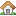Genetic calculator Version: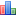4.0 final File size: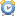14.3 Mb Language:En / Ru License: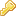Free OS: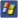Win XP/Vista/7/8

## How to solve genetic linkage problems

In the end of nineteenth century as a result of technological progress was be significantly increased the optical characteristics of microscopes, and was also significantly improved cytological research methods. This allowed scientists to make a series of important discoveries. Has been proven a leading role of cell's nucleus in the transmit of hereditary traits. They drew attention to the striking similarity between the behavior of chromosomes during the formation of gametes and fertilization, and scheme of inheritance of genetic factors, that Mendel described. On the basis of these data has been formulated the chromosome theory of inheritance. According to this theory a pair of factors localized in a pair of homologous chromosomes, and each of these chromosomes is carrier of one factor. Later, the term factor, which mean the basic unit of heredity, has been replaced by the term - gene. Thus we can say that genes, that located in the chromosomes, is the physical unit, through which the hereditary traits transmitted from parents to offspring. Each gene is represented in homologous chromosomes as a pair of alleles, which located in one locus, which means in the same place in these chromosomes. Now it was possible to explain the basic laws of inheritance in terms of the chromosome theory, as the characteristics of chromosomes motions during meiosis. Segregation of homologous chromosomes that occurs during anaphase 1 of meiosis and random distribution of alleles between the gametes is the basis for explanation of first law - Law of Segregation. And the independence of the segregation nonhomologous chromosomes during anaphase 1 of meiosis is the basis of the second law - the Law of Independent Assortment.

However, it is absolutly clear that every organism has a large number of traits and this quantity can be considerably greater than the number of chromosomes in haploid set. This is especially noticeable for species with a small number of chromosomes. For example number of chromosomes in haploid set in pea equal to 7, in rye is also equal to 7, fruit fly 4, and in roundworm 1. Then it is obvious, that in each chromosome must be located genes that determine the development at least a few different traits. Such genes are called linked and the number of linkage groups is equal to the number of chromosomes in the haploid set. Accordingly, these genes don't have to obey to the principle of an independent assortment - they must be inherited together as one unit.

In the genetic calculator, for designation of genetic linkage in the parental genotypes notation, the linked genes must be concluded in brackets. For dihybrid there are two possible localization of dominant and recessive alleles in the chromosomes. In the first case, the dominant alleles are localized in one of the pair of homologous chromosomes and recessive in the other - (AB)(ab). This variant of alleles localization is called cis-position. Genotype (AB) (ab) can be obtained from crosses of parents with genotypes (AB)(AB) - phenotype AB and (ab)(ab) - phenotype ab. In the second case the dominant and recessive alleles of a gene localized in different homologous chromosomes - (Ab)(aB). This variant of localization is called trans-position. Genotype (Ab) (aB), respectively, can be obtained from crosses of parents with genotypes (Ab)(Ab) - phenotype Ab and (aB)(aB) - phenotype aB. The difference in the ratio of phenotypes in the offspring for Mendelian inheritance and genetic linkage can demonstrated in the test crossing. In this crossing the number of types of gametes is equal to the number of phenotypic classes in the progeny. In the case of independent inheritance the genotype AaBb will give the four types of gametes AB, Ab, aB and ab with the ratio 1:1:1:1. In the case of a genetic linkage genotype (AB)(ab) can give only two types of gametes (AB) and (ab). Accordingly, by crossing the individuals with the genotype (AB)(ab) and (ab)(ab), we obtain two classes of phenotypes AB and ab with the ratio 1:1. Genotype (Ab)(aB) will also give two types of gametes (Ab) and (aB). And by crossing parents with genotypes (Ab) (aB) and (ab) (ab), we also obtain two classes of phenotypes Ab and aB with ratio 1:1. As you can see in both cases of genetic linkage. the phenotypic classes of offspring depends on the location of dominant and recessive alleles in the chromosomes and identical with the phenotypes of parents, by crossing which was obtained the original dihybrid.

But such results can be obtained only in the case of complete linkage. Typically, complete linkage is quite rare. The fact is that during meiosis, homologous chromosomes can exchange of regions with each other. This process is called crossing-over or genetic recombination. In the process of genetic recombination, the alleles, which located in linkage group in the parents, can segregate and give the new combinations in the gametes. Phenotypes, which are obtained from these gametes are called recombinants or crossovers. Thus, the progeny will be not two but four phenotype, as in the independent inheritance. But for linked inheritance the ratio will be different. Classes with the parental phenotypes will be form the bigger part of offspring, and the recombinant classes - smaller part. For example, for the genotype (AB)(ab) will be more offspring with phenotypes AB and ab and less with phenotypes Ab and aB, and for genotype (Ab) (aB) vice versa. The exact phenotype ratio will depend on the distance between genes. The farther away from each other located linked genes, then greater the probability, that crossing-over occurs between them. Thus, the frequency of crossing-over or recombination can be a measure for determination of the distance between genes. If the single crossing-over occur between genes and we known the amount of crossovers, then the distance between the genes can be calculated by the formula: RF = (c/t) * 100%, where the RF - the recombination frequency (the distance between genes), c - the amount of crossovers, t - the total amount of offspring, obtained from test cross. If the value of crossing-over exceeds 50%, then we can talk about an independent assortment of genes, that is without linkage inheritance. In the examples that you see below, we will use the Crossing Over Map Calculator to calculate the distance between genes and the Genetic Calculator for modeling genetic crosses with genetic linkage. It is important to note that Crossing Over Map Calculator can give correct results only for test crosses. First, let's look at some of the features of this calculator.

### Crossing Over Map Calculator

Crossing Over Map Calculator have a pretty simple and clear interface. It can be divided into two parts - in the part "Genes and phenotypes" you can enter the required data, and in the right - "Results" is shown the results of the calculations. You can find the radio buttons "Three Genes / Two Genes" in the left side. Since we are going to consider examples for two linked genes, then you should switch it to "Two Genes". And the specifications of genetic problems solution for the three linked genes will be considered later. Algorithm for data entry will look like this:
1) Choose the number of genes (in this case - switch the Radio Button in "Two Genes").
2) Write the dominant and recessive alleles of genes in the first table.
3) Press the "Calculate Phenotypes" button. The program automatically fills the first column in the second table by all possible combinations of phenotypes.
4) In the second column you will need write the number of individuals of each phenotype.
5) Press the "Calculate Results" button.

For examples with the two linked genes you can get the following results on the right side:
1) In the first table you can see the recombination frequency between genes. 1% recombination corresponds to obtaining one recombinant on 100 individuals. (1% recombination frequency = 1 m.u. (genetic map unit) unit of the genetic map, or a centimorgan (cM)).
2) Map Distance ( m.u.) - the actual distance between genes. This distance takes into account the effect of interference and possible double crossovers. The recombination frequency and Map distance are linked with each other by this formula ( Haldane’s Mapping Function ) : RF = (1 - e ^ ( -2 * map distance )) / 2 or map distance = - ( ln(1 - 2RF) / 2 ), where RF - the recombination frequency.
3) Parents genotypes. Based on the parental genotypes you can judge about genes localization - is they in cis- or trans- position.

#### Two linked genes in dihybrid test cross of tomato

Now, let's move to the concrete examples. In tomato genes that determine the height of the plants - T (tall) and t (dwarf) and the shape of the fruit - S (round) and s (pear-shaped ), located in one chromosome, ie they are linked. If we cross the homozygous plants with genotypes TTSS and ttss, it is also, as in the case of independent assorment, all the offspring will have the same phenotype. In this case, all the plants were high, with rounded fruits and have genotype TtSs. As a result of test cross, when these plants are crossed with homozygous recessive plants (ttss), was be obtained in the offspring 40 tall plants with round fruits, 40 dwarf plants with pear-shaped fruits, 10 tall plants with pear-shaped fruits, and 10 dwarf plants with round fruits. If the genes linkage was be complete, then in the offspring would be only tall plants with round fruits and dwarf plants with pear-shaped fruits in equal proportions, and if the genes were not linked, then the ratio of phenotypes would be 1:1:1:1. Thus we can say that in this case between the linked genes occurs crossing over, which gives a new recombinant phenotypes. Now let's analyze the obtained data.

Recombination calculation.

• Toggle Radio Button in "Two Genes".
• Write the dominant and recessive alleles of genes in the first table:
 T t S s
• Get the combination of phenotypes in the first column of the second table and write the amount of plants for each phenotype:
 TS 40 Ts 10 tS 10 ts 40
• Push the button "Calculate Results" and get results.

So let's see what we have:

• In the first place in the "Parentes genotypes" we see parental genotypes (non-crossover genotypes);
(TS)(ts)
(ts)(ts)
• Accordingly, our crossover genotypes is:
(Ts)(ts)
(tS)(ts)
• Second in the first table of the results we see recombination frequency = 20%. This is the distance between genes. The reliability of results you can check by the formula, that described above: RF = (c/t) * 100%. RF = ((10 + 10)/(40 + 40 + 10 + 10)) * 100% = (20/100) * 100% = 0.2 * 100% = 20%. Thus we can say that genes are localized in the trans-position at a distance of 20 santimorgan (centimorgan) (cM). Using the recombination frequency the program calculates the actual distance between genes = 25.541281 m.u. (In the field "Map distance")

Genetic calculation.

Now let's simulate this cross in genetic calculator. (TS)(ts) and (ts)(ts) - it's our parents genotypes. In these parents genotypes notations we must include the recombination frequency between genes - this value should be concluded in percent characters. There are three options for this entry:
1) (T%20%S)(ts)
2) (T%20%S)(t%20%s)
3) (TS)(t%20%s)

All three options are correct and you can use any of them. We will choose the first option and write the parents genotypes like this:
(T%20%S)(ts) x (t%20%s)(ts)

As a result of this crossing, we get phenotypes ratio 4 TS : 1 Ts : 1 tS : 4 ts or with probability 40% TS : 10% Ts : 10% tS : 40% ts. Since it is test cross, the ratio of gametes will be identical to the ratio of phenotypes.

#### Two linked genes in dihybrid test cross of maize

Consider another example of test cross. In maize genes that determine the color of seedlings - Green (dominant) and yellow (recessive) and brightness of the color of leaves - Opaque (dominant) and brigh(recessive), located in one chromosome. All plants from crosses of pure lines of maize have a Green seedlings and Opaque leaves. As a result of test cross, when these plants are crossed with homozygous recessive plants with yellow seedlings and brigh leaves, obtained in the progeny 240 plants with Green seedlings and Opaque leaves, 220 plants with yellow seedlings and brigh leaves, 36 plants with Green seedlings and brigh leaves, and 24 plants with yellow seedlings and Opaque leaves. Let's analyze the obtained data.

Recombination calculation.

• In the previous example, we marked alleles with a single letter. You probably noticed that it's not very comfortable, especially when you write the amount of individuals for each phenotype. But there is a better way, you can use the natural notations of traits for marking alleles. To do this, you need to conclude allele in these characters <Allell name>. So, we write the dominant and recessive alleles of genes in the first table in this way (Do not forget to switch the Radio Button in "Two Genes"):

• Get the combination of phenotypes in the first column of the second table and write the amount of plants for each phenotype:
 240 36 24 220
• Push the button "Calculate Results" and get results.
• In the field "Parentes genotypes" we see parental genotypes (non-crossover genotypes);
(<Green><Opaque>)(<yellow><bright>)
(<yellow><bright>)(<yellow><bright>)
• Accordingly, our crossover genotypes are:
(<Green><bright>)(<yellow><bright>)
(<yellow><Opaque>)(<yellow><bright>)
• In the first table of results we can see the recombination frequency = 11.5385% ( RF = (c/t) * 100%. RF = ((36 + 24)/(240 + 220 + 36 + 24)) * 100% = (60/520) * 100% = 0.115358 * 100% = 11.5385% ). Thus we can say that genes are localized in the trans-position at a distance of 11.5385 centimorgan (cM). Using recombination frequency the program calculates the actual distance between the genes = 13,118213 m.u. ( in the field "Map distance" )

Genetic calculation.

Now let's simulate the cross in genetic calculator. Write the parents genotypes:
(<Green>%11.5385%<Opaque>)(<yellow><bright>) and (<yellow>%11.5385%<bright>)(<yellow><bright>)

As a result of the cross we obtain such probabilities of phenotypes: 44.2308% <Green><Opaque> : 5.76925% <Green><bright> : 5.76925% <yellow><Opaque> : 44.2308% <yellow><bright>. Frequency of crossovers is approximately equal to 5.76925 + 5.76925 = 11.5385, and frequency of non-crossovers is approximately equal to 44.2308 + 44.2308 = 88.4616% ( or 100% - 11.5385% = 88.4615% ). If the total amount of individuals in the offspring is equal to 520, then amount of non-crossovers will be equal to 520 * 88.4616 / 100 = 460, and amount of crossovers 520 * 11.5385 / 100 = 60. In the experiment the amount of non-crossovers equal to 240 + 220 = 460, and amount of crossovers 36 + 24 = 60. Thus, the results is absolutely correct.

Random statistic

You can experiment with the possible variation in practical results of these crosses. "Random statistic" module of genetic calculator give you the opportunity to simulate the random distribution of progeny according to probabilities of phenotypes. Check the box "Generate statistic" and write the amount of the progeny (for the first cross- 100, for second - 520) in the field "Sample size". Each time you press the button "Calculate results" you'll get a new variant of assortment.

#### Two linked genes in dihybrid cross of Garden pea

To calculate the distance between the genes we must use only the results of test the crosses. But with Genetic calculator, we can simulate genetic linkage also for another crosses. Let's see this on example of a garden pea. Garden pea, rightly, we can call - the first object of genetic studies, as this plant the Gregor Mendel used in his experiments. Exactly on the basis of these experiments, he formulated the basic laws of genetics. As you know, in the pea the number of chromosomes in haploid set equal to 7, and of course, we can say that Gregor Mendel was lucky to choose to study the inheritance such pairs of traits, the genes of which was not linked, that's mean it was located in different chromosomes. In pea genes that determine the color of the flower - Purple (dominant) and red (recessive) and the shape of pollen grains - Long (dominant) and round (recessive), located in one chromosome at a distance of 12 centimorgan. All the offspring from crosses between plants with purple flowers and long pollen and plants with red flowers and round pollen had purple flowers and long pollen. As a result of self-pollination of these hybrids in the offspring was be obtained 69.5% plants with purple flowers and long pollen, 19.3% with red flowers and round pollen, and 5.6% plants with purple flowers and round pollen and 5.6%, with red flowers and long pollen. If the linkage was be complete, then the ratio of the progeny was be approximately equal to 3 : 1, like for monohybrid crosses, and if the genes was not be linked, then the phenotypes ratio in the case of independent inheritance of traits for dihybrid crossing was be 9 : 3 : 3 : 1. In this case, the ratio of non-recombinant phenotypes really approximately equal to 3 : 1, and we have a small amount of recombinant phenotypes.

Genetic calculation.

Now let's simulate the cross in genetic calculator. Write the parents genotypes:
(<Purple>%12%<Long>)(<red><round>) and (<Purple>%12%<Long>)(<red><round>).

As a result, we obtain our ratio of phenotypes: 69.5% <Purple><Long> : : 5.6% <Purple><round> : 5.6% <red><Long> : 19.3% <red><round>.

### Several Linkage Groups of Genes

Using Genetic calculator we can solve the problem also with several linkage groups. A good demonstration of this possibility will be solving the following example: In maize recessive genes, which determine to the curly leaves (cr) and dwarfism (d), are localized in the third chromosome at a distance of 18 map units (18%), and the dominant genes of rust resistance (Rp) (rust) and narrow leaves (Nl) - in the tenth chromosome at a distance of 24 map units (24%). We have a plant heterozygous by all genes, that are in the cis-position. Define: 1) What types of gametes, and with what probability can form this plant? 2) With what probability in the offspring we can expect the formation of homozygous dwarf plants with resistance to rust and with normal leaves?

Genetic calculation.

We can write the parents genotypes of our plants as follows: (<Cr>%18%<D>)(<cr><d>)(<Rp>%24%<Nl>)(<rp><nl>). To answer on first question we need to choose the - - "Gametes genotype 1" for type results and calculate it. In the second question we need to find the probability of the genotype - <Cr><Cr><d><d><Rp><Rp><nl><nl>. Change the type of results to "Genotypes" and go to the tab "Find". Click on each cell in the column "Combinations" and from the drop down list select a value that you need. For the first cell is <Cr><Cr><d><d>, and for the second - <Rp><Rp><nl><nl>. Push the "Find" button and you'll get an answer to the second question (0.011664).

### Crossing Over Mapping

Chromosomes - have a linear structure, and respectively the genes in the chromosomes are also located in the linear order. Thus, the frequency of crossing-over or genetic recombination between the genes can be used not only to determine the distance, but also to determine the relative position of these genes in the chromosome. However, you must know, that recombination have random character and between genes can also occur the double crossing-over. And often it's difficult to estimate the true distance between the genes, that located far enough from each other, because he is not always detected. As a result, the crossing-over frequency between the outermost genes is less than expected and not equal to the sum of the frequencies of single crossing-overs. Only the presence of the third gene between studied genes (called marker gene) allows you to accurately find the distance and positions of genes. As evidence we can examine the example of trihybrid crossing.

#### Three linked genes in trihybrid test cross of maize

In maize the genes, that determine the color of seedlings - Green (dominant) and yellow (recessive), the brightness of the leaves color - Opaque (dominant) and bright (recessive) and the shape of the leaves - cutting (recessive) and normal (dominant), are located in one chromosome. All plants from crosses of pure lines of maize have a green seedlings and opaque leaves with normal shape. As a result of test cross, when these plants are crossed with homozygous recessive plants with yellow seedlings and bright cutting leaves, was obtained the offspring:
270 plants with green seedlings and opaque leaves with the normal form
235 plants with yellow seedlings and bright cutting leaves
62 plants with green seedlings and bright cutting leaves
60 plants with yellow seedlings and opaque leaves with the normal form
48 plants with green seedlings and opaque cutting leaves
40 plants with yellow seedlings and bright leaves with the normal form
4 plants with green seedlings and bright leaves with the normal form
7 plants with yellow seedlings and opaque cutting leaves
Let's analyze the obtained data.

Recombination calculation.

• In Crossing Over Map Calculator switch the Radio Button to the "Three genes" and write the dominant and recessive alleles of genes in the first table in this way:

• We get the combination of phenotypes in the first column of the second table and write the amount of plants for each phenotype:
 270 48 4 62 60 7 40 235
• Push the button "Calculate Results" and get results.
• First of all this is Genes localization map (the order of genes in chromosome):
<yellow>-<bright>-<cutting> - in that order, these genes are located in the chromosome. Recombination frequency between <yellow>-<bright> equal to 18.3196%(Crossing over frequency), between <bright>-<cutting> is 13.6364%(Crossing over frequency) and between <yellow>-<cutting> it's equal to 31.9559%(Crossing over frequency).
• From the obtained eight classes of phenotypes - two is non-crossovers and they phenotypically identical to the parents phenotypes, and six is crossovers, two of which is double crossovers.
1. In the field "Parental genotypes" we see parents genotypes (non-crossovers genotypes);
(<Green><Opaque><Normal>)(<yellow><bright><cutting>)
(<yellow><bright><cutting>)(<yellow><bright><cutting>)
2. Becouse in the localization map the gen of leaves coloration (<bright>/<Opaque>) located in the middle, then the Double Crossovers is ( the lowest phenotypes ) :
7 <yellow><Opaque><cutting>
4 <Green><bright><Normal>
3. First pair of single crossovers is:
48 <Green><Opaque><cutting>
40 <yellow><bright><Normal>
4. Second pair of single crossovers is:
62 <Green><bright><cutting>
60 <yellow><Opaque><Normal>

Let's check this results by the our formula: RF = (c/t) * 100%. For first pair of single crossovers( Recombination frequency between gens - <bright>-<cutting> ): RF = ((48 + 40)/(235 + 270 + 7 + 4 + 48 + 40 + 62 + 60)) * 100% = (88/726) * 100% = 0.121212 * 100% = 12.1% For second pair of single crossovers( Recombination frequency between gens - <yellow>-<bright>): RF = ((62 + 60)/726) * 100% = 0.168044 * 100% = 16.8%. So we can expect the recombination frequency between gens <yellow>-<cutting> equal to 16.8% + 12.1% = 28.9%. However, from cross is obtained a different results. The frequency of single crossing-over between gens <yellow>-<cutting> = 28,9%, which is on 3% less than expected. The contradiction between the theoretically expected and practically obtained results is eliminated if we take into account the double crossing-over between genes <yellow>-<cutting>. Recombination frequency - RF = ((7 + 4)/726) * 100% = 0.015151 * 100% = 1.5%. So recombination frequency between gens - <bright>-<cutting> must be 12.1% + 1.5% = 13.6% and recombination frequency between gens - <yellow>-<bright> must be 16.8% + 1.5% = 18.3%. Therefore recombination frequency between gens <yellow>-<cutting> will be 13.6% + 18.3% = 31.9%. The distance between genes with the presence of double crossing-over is equal to the sum of percentage of the single crossing-overs and a doubled of percentage of double crossing-overs. In our example the distance between genes <yellow>-<cutting> is : 16.8% + 21.1% + 1.5% * 2 = 31.9%.

Genetic calculation.

Now let's simulate the cross in genetic calculator. Write the parents genotypes:
(<Green>%18.3196%<Opaque>%13.6364%<Normal>)(<yellow><bright><cutting>) and (<yellow><bright><cutting>)(<yellow><bright><cutting>)

As a result of this crossing, we obtain such phenotypes probabilitys:

 35.2711% 5.56913% 1.24907% 7.91073% 7.91073% 1.24907% 5.56913% 35.2711%

The frequency of double crossing-over is approximately equal to 1.24907 + 1.24907 = 2.49814%. The frequency of single crossovers is approximately equal to 5.76925 + 5.76925 = 11.5385 + 2.49814 = 13.6364% and 7.91073 + 7.91073 = 15.82146 + 2.49814 = 18.3196%. Accordingly, the total frequency of crossovers is 13.6364 + 18.3196 = 31.956%. Thus, the results correspond to the experimental and absolutely correct.